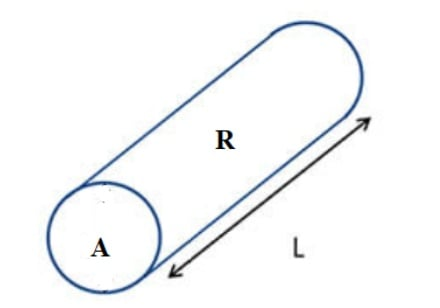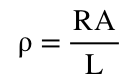# Resistivity Calculator

 Resistance Ohm Kilo Ohm Mega Ohm Area m2 Length m

### OUTPUT

 Resistivity Ω∙m

#### How to Calculate Resistivity of A Component like Wire or Coil?

The Resistivity Calculator allows use to easily calculate the resistivity of a component like a wire or coil, based on its resistance value, its length, and its cross-sectional area.

Resistivity, unlike resistance, is a measure of the resistance of a given size of a particular material, or in basic terms the resistivity of a material is the amount of resistance it may provide to a current depending on its dimensions. The resistivity of any material directly depends on the material’s cross-sectional area. The greater the cross-sectional area of a material, the greater the resistivity will be. Likewise, the smaller the cross-sectional area, the lower the resistivity will be. In addition to area, the resistivity also depends directly on the resistance of the material. The resistivity of a material is inversely proportional to the length of the material. The greater the length of the material, the lower the resistivity and the smaller the length of the material, the greater the resistivity.EquationWhere:

Resistivity (ρ) = resistivity of the material in ohm-m (Ω-m)

Resistance (R) = resistance of the material in ohms

Length (L) = length of the material in meters

Area (A) = cross-sectional area of the material in square meters (m2)